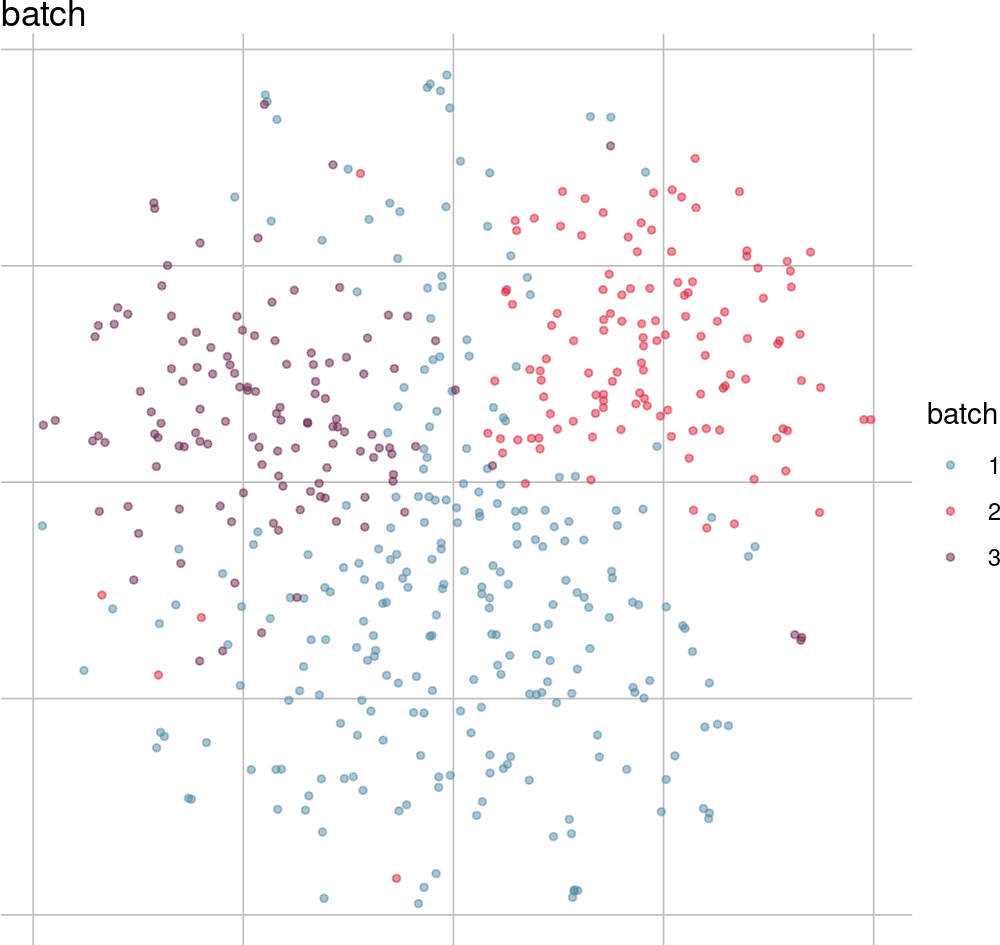# 1 Introduction

The CellMixS package is a toolbox to explore and compare group effects in single-cell RNA-seq data. It has two major applications:

• Detection of batch effects and biases in single-cell RNA-seq data.
• Evaluation and comparison of data integration (e.g. after batch effect correction).

For this purpose it introduces two new metrics:

• Cellspecific Mixing Score (CMS): A test for batch effects within k-nearest neighbouring cells.
• Local Density Differences (ldfDiff): A score describing the change in relative local cell densities by data integration or projection.

It also provides implementations and wrappers for a set of metrics with a similar purpose: entropy, the inverse Simpson index (Korsunsky et al. 2018), and Seurat’s mixing metric and local structure metric (Stuart et al. 2018). Besides this, several exploratory plotting functions enable evaluation of key integration and mixing features.

# 2 Installation

CellMixS can be installed from Bioconductor as follows.

``````if (!requireNamespace("BiocManager"))
install.packages("BiocManager")
BiocManager::install("CellMixS")``````

After installation the package can be loaded into R.

``library(CellMixS)``

# 3 Getting started

CellMixS uses the `SingleCellExperiment` class from the SingleCellExperiment Bioconductor package as the format for input data.

The package contains example data named sim50, a list of simulated single-cell RNA-seq data with varying batch effect strength and unbalanced batch sizes.

Batch effects were introduced by sampling 0%, 20% or 50% of gene expression values from a distribution with modified mean value (e.g. 0% - 50% of genes were affected by a batch effect).

All datasets consist of 3 batches, one with 250 cells and the others with half of its size (125 cells). The simulation is modified after (Büttner et al. 2019) and described in sim50.

``````# Load required packages
suppressPackageStartupMessages({
library(SingleCellExperiment)
library(cowplot)
library(limma)
library(magrittr)
library(dplyr)
library(purrr)
library(ggplot2)
library(scater)
})``````
``````# Load sim_list example data
package = "CellMixS"))
names(sim_list)
#>  "batch0"  "batch20" "batch50"

sce50 <- sim_list[["batch50"]]
class(sce50)
#>  "SingleCellExperiment"
#> attr(,"package")
#>  "SingleCellExperiment"

table(sce50[["batch"]])
#>
#>   1   2   3
#> 250 125 125``````

## 3.2 Visualize batch effect

Often batch effects can already be detected by visual inspection and simple visualization (e.g. in a normal tSNE or UMAP plot) depending on the strength. CellMixS contains various plotting functions to visualize group label and mixing scores aside. Results are `ggplot` objects and can be further customized using ggplot2. Other packages, such as scater, provide similar plotting functions and could be used instead.

``````# Visualize batch distribution in sce50
visGroup(sce50, group = "batch")````````````# Visualize batch distribution in other elements of sim_list
batch_names <- c("batch0", "batch20")

vis_batch <- lapply(batch_names, function(name){
sce <- sim_list[[name]]
visGroup(sce, "batch") + ggtitle(paste0("sim_", name))
})

plot_grid(plotlist = vis_batch, ncol = 2)``````